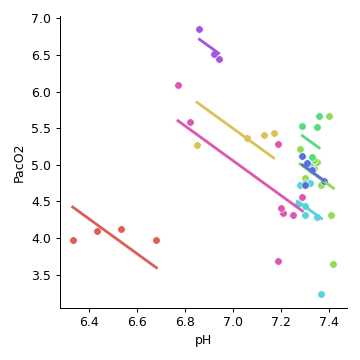# pingouin.rm_corr

pingouin.rm_corr(data=None, x=None, y=None, subject=None)[source]

Repeated measures correlation.

Parameters
datapandas.DataFrame

Dataframe.

x, ystring

Name of columns in data containing the two dependent variables.

subjectstring

Name of column in data containing the subject indicator.

Returns
statspandas.DataFrame
• 'r': Repeated measures correlation coefficient

• 'dof': Degrees of freedom

• 'pval': p-value

• 'CI95': 95% parametric confidence intervals

• 'power': achieved power of the test (= 1 - type II error).

Notes

Repeated measures correlation (rmcorr) is a statistical technique for determining the common within-individual association for paired measures assessed on two or more occasions for multiple individuals.

Rmcorr accounts for non-independence among observations using analysis of covariance (ANCOVA) to statistically adjust for inter-individual variability. By removing measured variance between-participants, rmcorr provides the best linear fit for each participant using parallel regression lines (the same slope) with varying intercepts. Like a Pearson correlation coefficient, the rmcorr coefficient is bounded by − 1 to 1 and represents the strength of the linear association between two variables.

Results have been tested against the rmcorr R package.

Missing values are automatically removed from the dataframe (listwise deletion).

Examples

>>> import pingouin as pg

Now plot using the pingouin.plot_rm_corr() function:
>>> import pingouin as pg